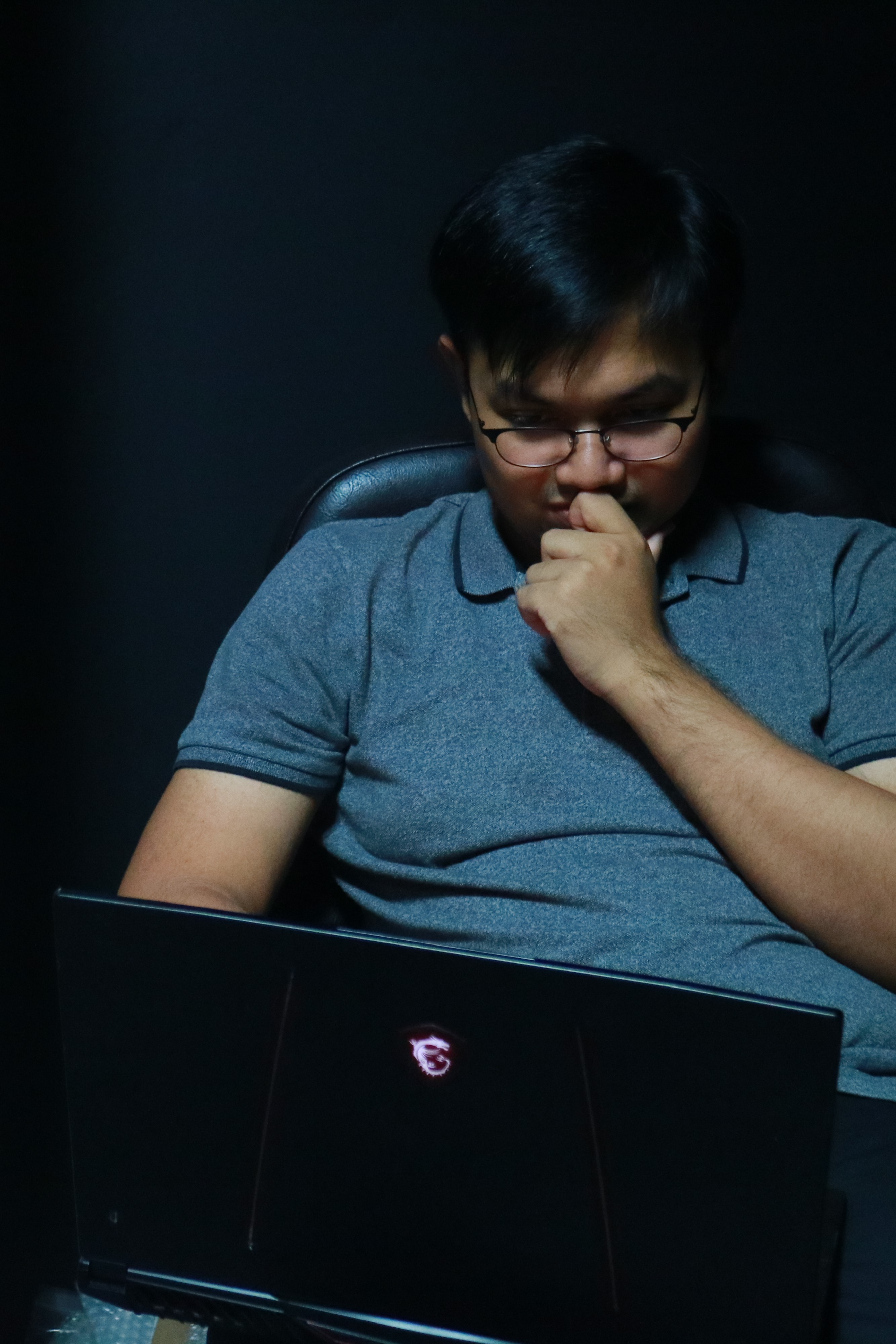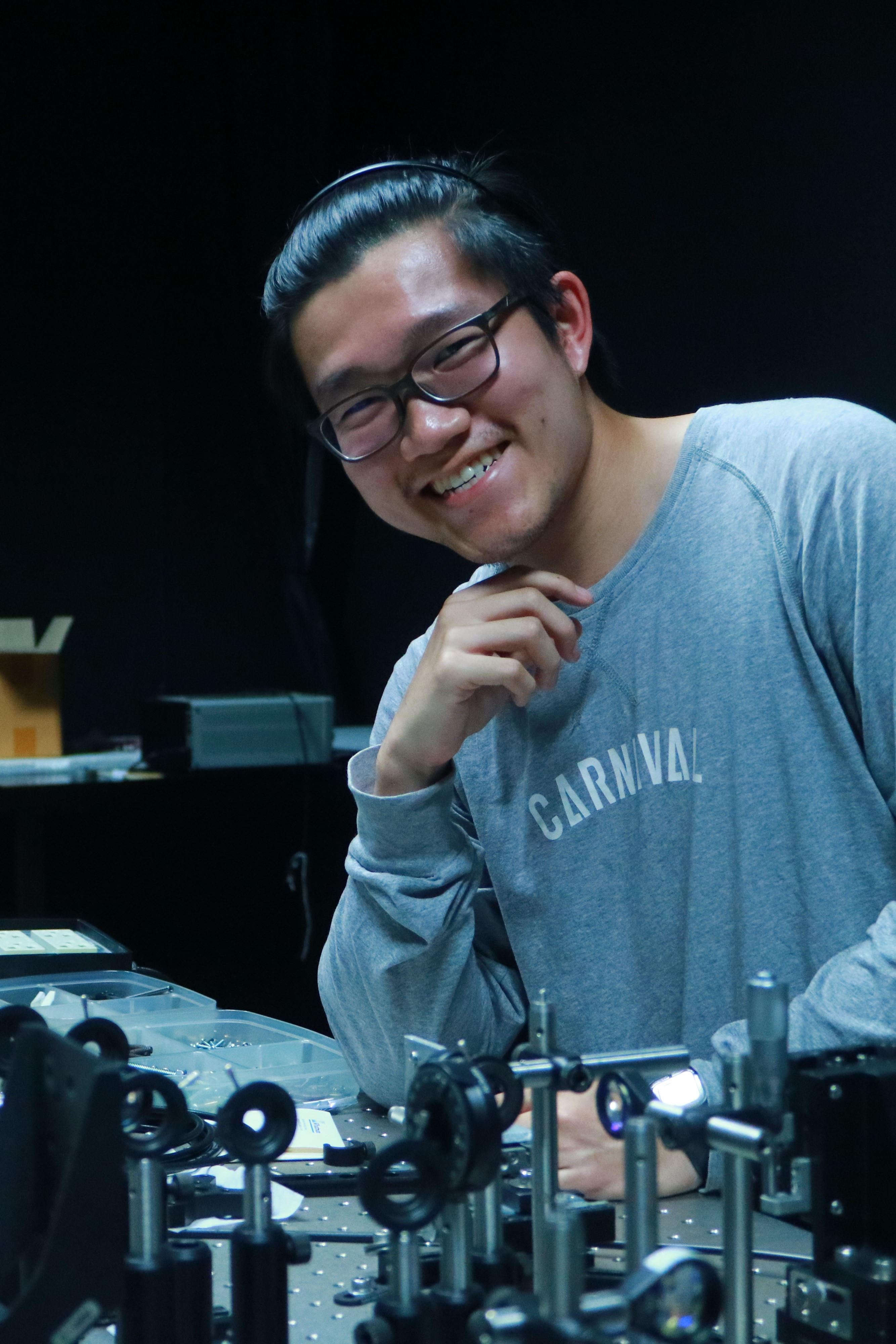# Our work is on Quantum Open system

An open quantum system is now an essential topic for this decade since it typically associates with the development of quantum computers, e.g. quantum measurement in superconducting qubit experiments. In general, a closed quantum system can be described by a unitary evolution. However, in practical, we cannot avoid to face with an open quantum system which it can no longer described by a unitary evolution, it then gives rise to the decoherence. The decoherence is now the most radical problem of an open quantum system since such that system can no longer preserve the quantumness. Quantum decoherence can be thought as leaking of some information to the environment (e.g. system energy) which can reach a complicated understanding of quantum system. At the present time, there are many researcher working on an open quantum system, especially, our group, we are focussing on quantum measurement, quantum trajectory estimation and so on.##### THEORY

An open quantum system is now an essential topic for this decade since it typically associates with the development of quantum computers, e.g. quantum measurement in superconducting qubit experiments. In general, a closed quantum system can be described by a unitary evolution. However, in practical, we cannot avoid to face with an open quantum system which it can no longer described by a unitary evolution, it then gives rise to the decoherence. The decoherence is now the most radical problem of an open quantum system since such that system can no longer preserve the quantumness. Quantum decoherence can be thought as leaking of some information to the environment (e.g. system energy) which can reach a complicated understanding of quantum system. At the present time, there are many researcher working on an open quantum system, especially, our group, we are focussing on quantum measurement, quantum trajectory estimation and so on.##### COMPUTING

An open quantum system is now an essential topic for this decade since it typically associates with the development of quantum computers, e.g. quantum measurement in superconducting qubit experiments. In general, a closed quantum system can be described by a unitary evolution. However, in practical, we cannot avoid to face with an open quantum system which it can no longer described by a unitary evolution, it then gives rise to the decoherence. The decoherence is now the most radical problem of an open quantum system since such that system can no longer preserve the quantumness. Quantum decoherence can be thought as leaking of some information to the environment (e.g. system energy) which can reach a complicated understanding of quantum system. At the present time, there are many researcher working on an open quantum system, especially, our group, we are focussing on quantum measurement, quantum trajectory estimation and so on.##### EXPERIMENT

An open quantum system is now an essential topic for this decade since it typically associates with the development of quantum computers, e.g. quantum measurement in superconducting qubit experiments. In general, a closed quantum system can be described by a unitary evolution. However, in practical, we cannot avoid to face with an open quantum system which it can no longer described by a unitary evolution, it then gives rise to the decoherence. The decoherence is now the most radical problem of an open quantum system since such that system can no longer preserve the quantumness. Quantum decoherence can be thought as leaking of some information to the environment (e.g. system energy) which can reach a complicated understanding of quantum system. At the present time, there are many researcher working on an open quantum system, especially, our group, we are focussing on quantum measurement, quantum trajectory estimation and so on.